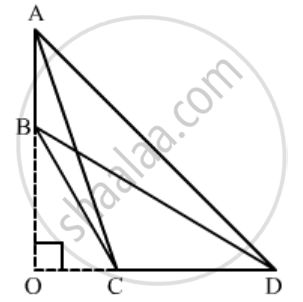Advertisement Remove all ads

# In a Quadrilateral Abcd, Given that ∠A + ∠D = 90°. Prove that Ac2 + Bd2 = Ad2 + Bc2. - Mathematics

Sum

In a quadrilateral ABCD, given that ∠A + ∠D = 90°. Prove that AC2 + BD2 = AD2 + BC2.

Advertisement Remove all ads

#### Solution

Given: A quadrilateral ABCD where A + D = 90°.

To prove: AC2 + BD2 = AD2 + BC2

Construction: Extend AB and CD to intersect at O.Proof:

In ΔAOD, A + O + D = 180°

⇒ ∠O = 90° [A + D = 90°]

Apply Pythagoras Theorem in ΔAOC and ΔBOD,

AC2 = AO2 + OC2

BD2 = OB2 + OD2

∴ AC2 + BD2 = (AO2 + OD2) + (OC2 + OB2)

⇒ AC2 + BD2 = AD2 + BC2

This proves the given relation.

Concept: Triangles Examples and Solutions
Is there an error in this question or solution?
Advertisement Remove all ads

#### APPEARS IN

RD Sharma Class 10 Maths
Chapter 7 Triangles
Q 31 | Page 127
Advertisement Remove all ads

#### Video TutorialsVIEW ALL 

Advertisement Remove all ads
Share
Notifications

View all notifications

Forgot password?
Course